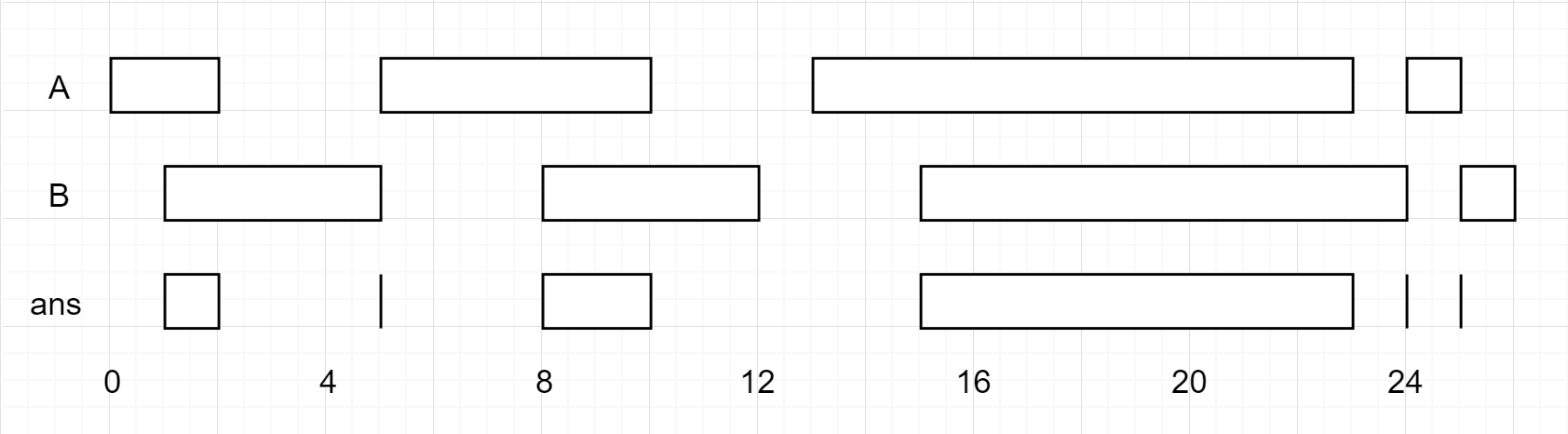# 区间列表的交集

## 986. 区间列表的交集 (Medium)```输入：firstList = [[0,2],[5,10],[13,23],[24,25]], secondList = [[1,5],[8,12],[15,24],[25,26]]

```

```输入：firstList = [[1,3],[5,9]], secondList = []

```

```输入：firstList = [], secondList = [[4,8],[10,12]]

```

```输入：firstList = [[1,7]], secondList = [[3,10]]

```

• `0 <= firstList.length, secondList.length <= 1000`
• `firstList.length + secondList.length >= 1`
• `0 <= starti < endi <= 109`
• `endi < starti+1`
• `0 <= startj < endj <= 109 `
• `endj < startj+1`

[数组] [双指针]

### 相似题目

1. 合并区间 (Medium)
2. 合并两个有序数组 (Easy)
3. 员工空闲时间 (Hard)